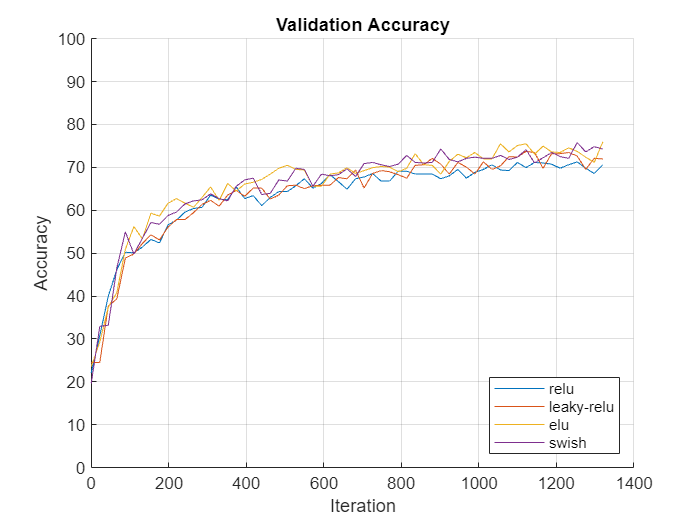Main Content

# Compare Activation Layers

This example shows how to compare the accuracy of training networks with ReLU, leaky ReLU, ELU, and swish activations layers.

Training deep learning neural networks requires using nonlinear activation functions such as the ReLU and swish operations. Some activation layers can result in better training performance at the cost of extra computation time. When training a neural network, you can try using different activation layers to see if training improves.

This example shows how to compare the validation accuracy of training a SqueezeNet neural network when using either ReLU, leaky ReLU, ELU, and swish activations layers and compares the accuracy given a validation set of images.

### Load Data

Download the Flowers data set.

```url = 'http://download.tensorflow.org/example_images/flower_photos.tgz'; downloadFolder = tempdir; filename = fullfile(downloadFolder,'flower_dataset.tgz'); dataFolder = fullfile(downloadFolder,'flower_photos'); if ~exist(dataFolder,'dir') fprintf("Downloading Flowers data set (218 MB)... ") websave(filename,url); untar(filename,downloadFolder) fprintf("Done.\n") end```

### Prepare Data for Training

Load the data as an image datastore using the `imageDatastore` function and specify the folder containing the image data.

```imds = imageDatastore(dataFolder, ... 'IncludeSubfolders',true, ... 'LabelSource','foldernames');```

View the number of classes of the training data.

`numClasses = numel(categories(imds.Labels))`
```numClasses = 5 ```

Divide the datastore so that each category in the training set has 80% of the images and the validation set has the remaining images from each label.

`[imdsTrain,imdsValidation] = splitEachLabel(imds,0.80,'randomize');`

Specify augmentation options and create an augmented image data store containing the training images.

• Randomly reflect the images in the horizontal axis.

• Randomly scale the images by up to 20%.

• Randomly rotate the images by up to 45 degress.

• Randomly translate the images by up to 3 pixels.

• Resize the images to the input size of the network (227-by-227)

```imageAugmenter = imageDataAugmenter( ... 'RandXReflection',true, ... 'RandScale',[0.8 1.2], ... 'RandRotation',[-45,45], ... 'RandXTranslation',[-3 3], ... 'RandYTranslation',[-3 3]); augimdsTrain = augmentedImageDatastore([227 227],imdsTrain,'DataAugmentation',imageAugmenter);```

Create an augmented image datastore for the validation data that resizes the images to the input size of the network. Do not apply any other image transformations to the validation data.

`augimdsValidation = augmentedImageDatastore([227 227],imdsValidation);`

### Create Custom Plotting Function

When training multiple networks, to monitor the validation accuracy for each network on the same axis, you can use the `OutputFcn` training option and specify a function that updates a plot with the provided training information.

Create a function that takes the information struct provided by the training process and updates an animated line plot. The `updatePlot` function, listed in the Plotting Function section of the example, takes as input the information structure and updates the specified animated line.

### Specify Training Options

Specify the training options:

• Train using a mini-batch size of 128 for 60 epochs.

• Shuffle the data each epoch.

• Validate the neural network once per epoch using the held-out validation set.

```miniBatchSize = 128; numObservationsTrain = numel(imdsTrain.Files); numIterationsPerEpoch = floor(numObservationsTrain / miniBatchSize); options = trainingOptions('adam', ... 'MiniBatchSize',miniBatchSize, ... 'MaxEpochs',60, ... 'Shuffle','every-epoch', ... 'ValidationData',augimdsValidation, ... 'ValidationFrequency',numIterationsPerEpoch, ... 'Verbose',false);```

### Train Neural Networks

For each of the activation layer types ReLU, leaky ReLU, ELU, and swish, train a SqueezeNet network.

Specify the types of activation layers.

`activationLayerTypes = ["relu" "leaky-relu" "elu" "swish"];`

Initialize the customized training progress plot by creating animated lines with colors specified by `colororder` function.

```figure colors = colororder; for i = 1:numel(activationLayerTypes) line(i) = animatedline('Color',colors(i,:)); end ylim([0 100]) legend(activationLayerTypes,'Location','southeast'); xlabel("Iteration") ylabel("Accuracy") title("Validation Accuracy") grid on```

Loop over each of the activation layer types and train the neural network. For each activation layer type:

• Create a function handle `activationLayer` that creates the activation layer.

• Create a new SqueezeNet network without weights and replace the activation layers (the ReLU layers) with layers of the activation layer type using the function handle `activationLayer`.

• Replace the final convolution layer of the neural network with one specifying the number of classes of the input data.

• Update the validation accuracy plot by setting the `OutputFcn` property of the training options object to a function handle representing the `updatePlot` function with the animated line corresponding to the activation layer type.

• Train and time the network using the `trainNetwork` function.

```for i = 1:numel(activationLayerTypes) activationLayerType = activationLayerTypes(i); % Determine activation layer type. switch activationLayerType case "relu" activationLayer = @reluLayer; case "leaky-relu" activationLayer = @leakyReluLayer; case "elu" activationLayer = @eluLayer; case "swish" activationLayer = @swishLayer; end % Create SqueezeNet layer graph. lgraph = squeezenet('Weights','none'); % Replace activation layers. if activationLayerType ~= "relu" layers = lgraph.Layers; for j = 1:numel(layers) if isa(layers(j),'nnet.cnn.layer.ReLULayer') layerName = layers(j).Name; layer = activationLayer('Name',activationLayerType+"_new_"+j); lgraph = replaceLayer(lgraph,layerName,layer); end end end % Specify number of classes in final convolution layer. layer = convolution2dLayer([1 1],numClasses,'Name','conv10'); lgraph = replaceLayer(lgraph,'conv10',layer); % Specify custom plot function. options.OutputFcn = @(info) updatePlot(info,line(i)); % Train the network. start = tic; [net{i},info{i}] = trainNetwork(augimdsTrain,lgraph,options); elapsed(i) = toc(start); end```Visualize the training times in a bar chart.

```figure bar(categorical(activationLayerTypes),elapsed) title("Training Time") ylabel("Time (seconds)")```In this case, using the different activation layers yield similar final validation accuracies with leaky ReLU and swish layers having slightly higher values. Using swish activation layers converge in fewer iterations. When compared to the other activaiton layers, using ELU layers makes the validation accuracy converge in more iterations and requires more computation time.

### Plotting Function

The `updatePlot` function takes as input the information structure `info` and updates the validation plot specified by the animated line `line`.

```function updatePlot(info,line) if ~isempty(info.ValidationAccuracy) addpoints(line,info.Iteration,info.ValidationAccuracy); drawnow limitrate end end```

Download ebook